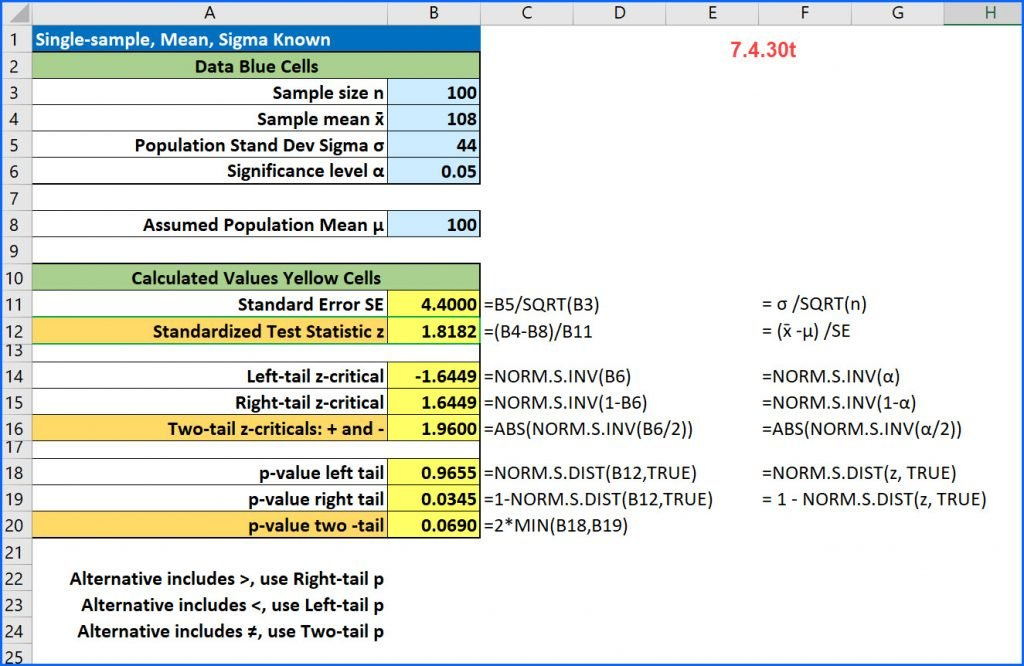# Single-sample z-test for the mean [7.4.30t]

Here is a common problem from intro stats: [7.4.30t]

A random sample of 100 observations from a population with a standard deviation of 44 yielded a sample mean of 108. Test the null hypothesis that μ = 100 against the alternative that μ > 100 at an alpha of 0.05.Here because the alternative contains the > math operation, this is a right tail test and the p-value is 0.035 which is less than alpha. The decision is to reject the null and conclude that at the 5% significance level there is enough evidence to support a claim that the mean is greater than 100.

Here is the StatCrunch solution:This site uses Akismet to reduce spam. Learn how your comment data is processed.